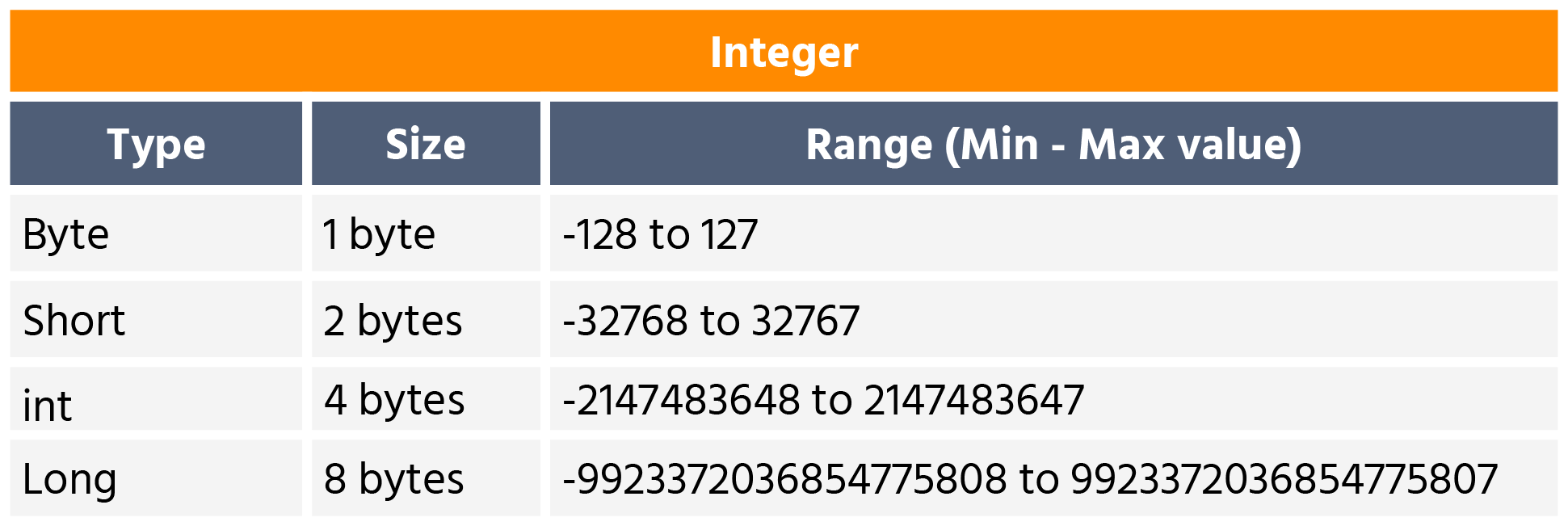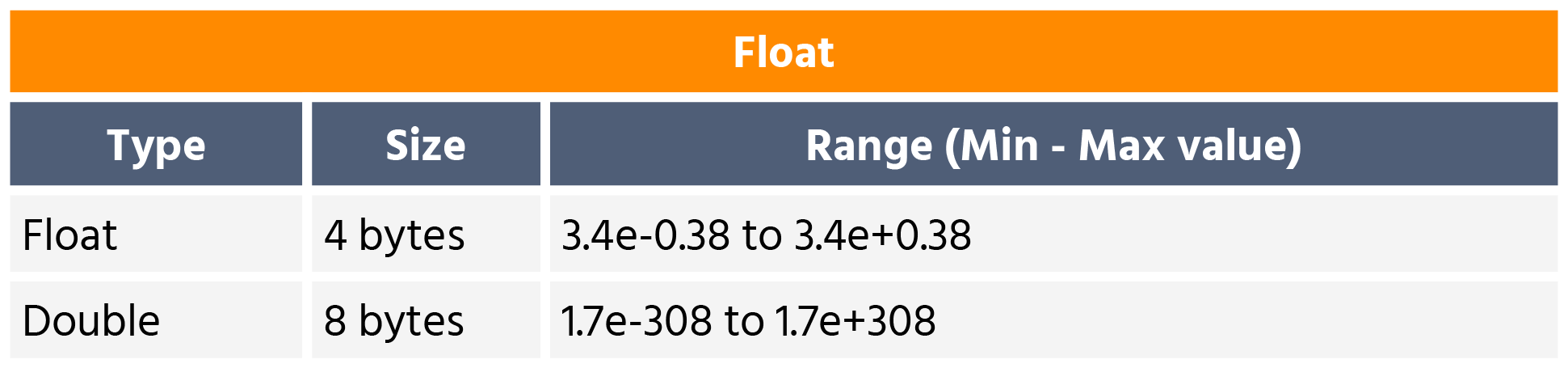Course Content

Introduction to JavaData Types

# Java Data types

A data type is an attribute of data that tells the computer what kind of data it is and how it should be processed. There are two types of data types in Java:

• primitive: int, byte, short, long, double, float, boolean, and char;
• non-primitive: arrays and classes.

## Integer type

Integer types are whole numbers that can be positive, negative, or zero. There are 4 integer types in Java:

• byte;
• short;
• int;
• long.## Floating point types

Floating point types represent real numbers as a fractional component and an exponent. A fractional component is a decimal number, and the exponent is an integer exponent that determines the position of the decimal point.

Floating point types in Java:

• float;
• double.

Representing single-precision and double-precision floating point numbers, respectively.## Character type

A character type is a data type that represents a character value.

A character value is a single 16-bit Unicode character. A character type stores any character from any language, including letters, digits, punctuation marks, and whitespace.

The basic character type is the char data type. The `char` is denoted by a single character in single quotes (`'s'`).

## Boolean type

Boolean data type represents one bit of information. It is used to store two values:

• `True`;
• `False`.Main.javaSection 1.

Chapter 2